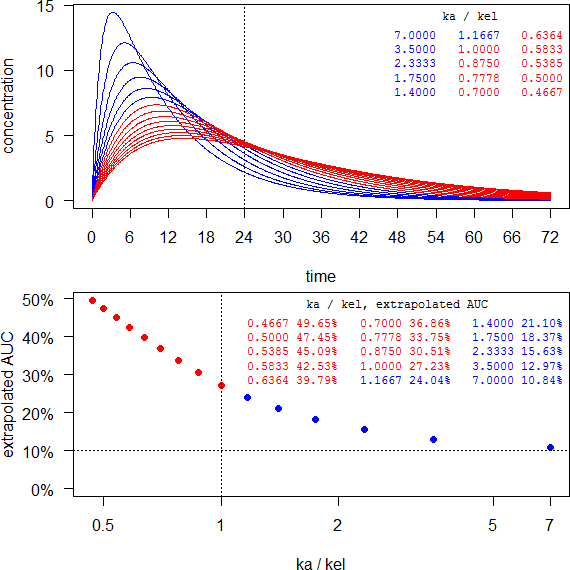EMA: Waiving MD study [Regulatives / Guidelines]

Dear all,

at the end anscript to play with.

For any elimination half life longer than ~7 hours and an intended dosing interval of 24 hours it will be impossible to get the MD study waived. Red are formulations with flip-flop PK (kakel).

last <- 72
ke   <- log(2)/7
tlag <- 0If the half life is short (say, 3 hours) you don’t have to dive deep into flip-flop PK and may succeed. However, such a limited extension might not match your development target.

last <- 36
ke   <- log(2)/3
tlag <- 1C.t <- function(f, D, V, ka, ke, t, tlag) { # 1-compartment
if (any(c(length(f) > 1, length(D) > 1, length(V) > 1,
length(ka) > 1, length(ke) > 1)))
stop("f, D, V, ka, ke have to be scalars.")
if (f <= 0 | f > 1) stop("f has to be positive <= 1.")
if (any(c(D <= 0, V <= 0, ka <= 0, ke <= 0)))
stop("D, V, ka, ke have to be positive.")
if (missing(tlag)) tlag <- 0
if (tlag < 0) stop("tlag has to be >= 0.")
if (ka != ke) {
C <- f*D*ka/(V*(ka-ke))*(exp(-ke*(t-tlag))-exp(-ka*(t-tlag)))
} else { # flip-flop PK
k <- ka
C <- f*D*k*(t-tlag)/V*exp(-k*(t-tlag))
}
C[C < 0] <- NA # negatives due to lag-time
return(C)
}
AUC.t <- function(C, t, tau) { # lin-up/log-down method
t <- t[t <= tau]             # for AUC until tau
AUC.t <- 0
for (j in 2:length(t)) {
if (!is.na(C[j-1])) {
if (C[j] >= C[j-1]) { # linear-up
AUC.t <- AUC.t+0.5*(t[j]-t[j-1])*(C[j]+C[j-1])
} else {              # logarithmic-down
AUC.t <- AUC.t+(t[j]-t[j-1])*(C[j]-C[j-1])/log(C[j]/C[j-1])
}
}
}
return(AUC.t)
}
AUC.i <- function(AUC.tau, C, t, last, tau) { # extrapolation
tlast.3  <- tail(t[t <= last], 3)
Clast.3  <- tail(C[t <= last], 3)
Clast    <- tail(Clast.3, 1)
Ctau     <- C[t == tau]
mod      <- lm(log(Clast.3) ~ tlast.3)
lambda.z <- -coef(mod)[]
if (lambda.z <= 0) stop("increasing concentrations")
AUC.inf  <- AUC.tau+Ctau/lambda.z
return(AUC.inf)
}
last  <- 72
tau   <- 24
f     <- 1
D     <- 100
V     <- 5
ke    <- log(2)/7       # moderate elimination half life
tlag  <- 0              # optional >= 0
forms <- 15             # no. of formulations
ka    <- log(2)/1:forms # vary absorption
t     <- seq(0, last, 0.1)
AUC.ext <- numeric()
windows(width = 6, height = 6)
par(mar = c(4, 4, 0.2, 0) + 0.1, family = "sans")
split.screen(c(2, 1))
screen(1)
for (j in 1:forms) {
C <- C.t(f, D, V, ka[j], ke, t, tlag)
if (j == 1) {
plot(t, C, type = "l", col = "blue", axes = FALSE,
xlab = "time", ylab = "concentration")
abline(v = tau, lty = 3)
axis(1, labels = seq(0, last, 6), at = seq(0, last, 6))
axis(2, las = 1)
} else {
if (ka[j] > ke) {
lines(t, C, col = "blue")
} else { # flip-flop
lines(t, C, col = "red")
}
}
AUC.tau    <- AUC.t(C, t, tau)
AUC.inf    <- AUC.i(AUC.tau, C, t, last, tau)
AUC.ext[j] <- (AUC.inf-AUC.tau)/AUC.inf
}
clr <- ifelse (ka/ke > 1, clr <- "blue", clr <- "red")
par(family = "mono")
legend("topright", title = "ka / kel", box.lty = 0,
legend = sprintf("%.4f", ka/ke), title.col = "black",
text.col = clr, cex = 0.75, ncol = 3); box()
screen(2)
par(family = "sans")
plot(ka/ke, 100*AUC.ext, ylim = c(0, max(100*AUC.ext)), log = "x",
axes = FALSE, xlab = "ka / kel", ylab = "extrapolated AUC",
pch = 19, col = clr)
points(rev(ka/ke)[which(rev(AUC.ext) <= 0.1)],
rev(100*AUC.ext)[rev(AUC.ext) <= 0.1], pch = 21, col = "#00C000",
bg = "transparent", cex = 1.55)
xticks <- c(axTicks(side = 1, log = TRUE), max(ka/ke))
axis(1, at = xticks, labels = xticks)
axis(2, las = 1, at = c(0, pretty(100*AUC.ext)),
labels = sprintf("%.0f%%", c(0, pretty(100*AUC.ext))))
abline(v = 1, lty = 3)  # flip-flop limit
abline(h = 10, lty = 3) # MD waiver limit
par(family = "mono")
legend("topright", title = "ka / kel, extrapolated AUC", box.lty = 0,
legend = sprintf("%.4f %.2f%%", rev(ka/ke), rev(100*AUC.ext)),
title.col = "black", text.col = rev(clr), cex = 0.75, ncol = 3,
bg = "white"); box()
close.screen(all = TRUE)
par(op)

Dif-tor heh smusma 🖖
Helmut SchützThe quality of responses received is directly proportional to the quality of the question asked. 🚮
Science QuotesIng. Helmut Schütz